# PSAT Math : How to find the solution to an equation

## Example Questions

1 2 3 4 6 Next →

### Example Question #56 : How To Find The Solution To An Equation

Ben is walking three dogs that weigh an average of 75 pounds each. Ben begins to walk a fourth dog, and the average weight of the dogs decreases to 70 pounds. What is the weight in pounds of the fourth dog?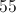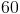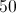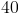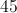Explanation:

The total weight of the first three dogs is 225 pounds. This amount, plus the weight of the fourth dog, divided by total number of dogs, is the new average weight: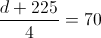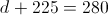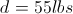Pets Plus makes bird houses.  Their monthly fixed expenses are $750. The cost for each bird house is$15.  The bird houses sell for $40. What is the monthly break-even point at Pets Plus? Possible Answers: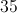Correct answer:Explanation: Let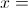the number of bird houses sold each month.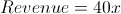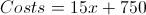The break-even point is where the revenue is the same as the costs: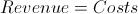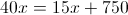Solve for: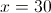Therefore, Pets Plus must sell 30 bird houses to break-even. ### Example Question #58 : How To Find The Solution To An Equation Pets Plus makes bird houses. Their monthly fixed expenses are$750.  The cost for each bird house is $15. The bird houses sell for$40.

If Pets Plus sells 50 bird houses, what is the profit?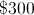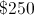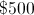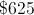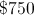Explanation:

Let= the number of birdhouses sold each month.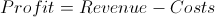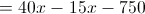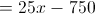Substituting in 50 forgives an answer of 500, so the profit on 50 birdhouses is \$500.

### Example Question #91 : How To Find The Solution To An Equation

George is three times older than Joey.  The sum of their ages is 16.  What is the product of their ages?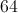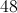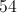Explanation:

Let= Joey's age and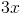= George's age.

Then the equation to solve becomes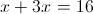.Therefore, Joey is 4 years old and George is 12 years old, so the product of their ages is 48.

### Example Question #91 : How To Find The Solution To An Equation

Three consecutive even numbers add to 42.  What is the middle number?Explanation:

Let= 1st even number,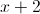= 2nd even number, and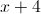= 3rd even number.

Then the equation to solve becomes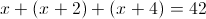.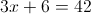Thus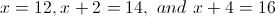, so the middle number is 14.

### Example Question #151 : Algebra

Consider the following equation: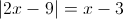Which of the following must be true?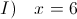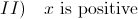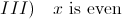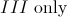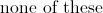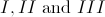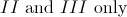Explanation:

The quantity inside the absolute value brackets must equal either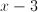or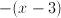, depending on whether the quantity inside the brackets is positive or negative. We therefore have two seperate equations: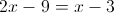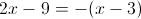To solve the first equation, add 9 to both sides:Subtractfrom both sides: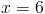This is the first solution. Now let's look at the second equation. The distributive law gives us: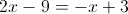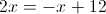Addto both sides: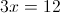Divide both sides by 3: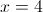Therefore,is either 4 or 6.

Statement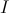does NOT have to be true becausecan also equal 4.

Statement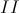must be true because both 4 and 6 are positive .

Finally, statement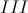always holds because 4 and 6 are both even.

### Example Question #61 : Linear / Rational / Variable Equations

What property of arithmetic is demonstrated here?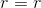Commutative

Reflexive

Identity

Symmetric

Transitive